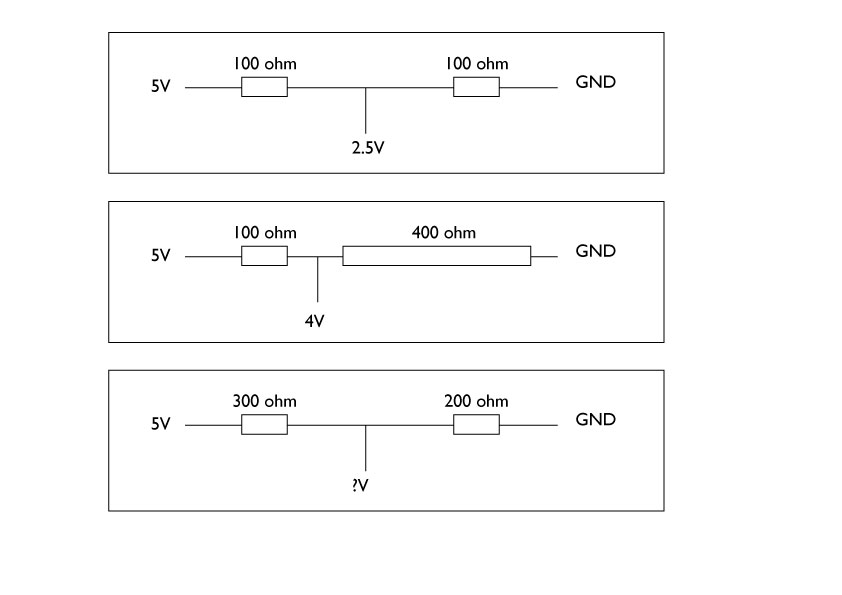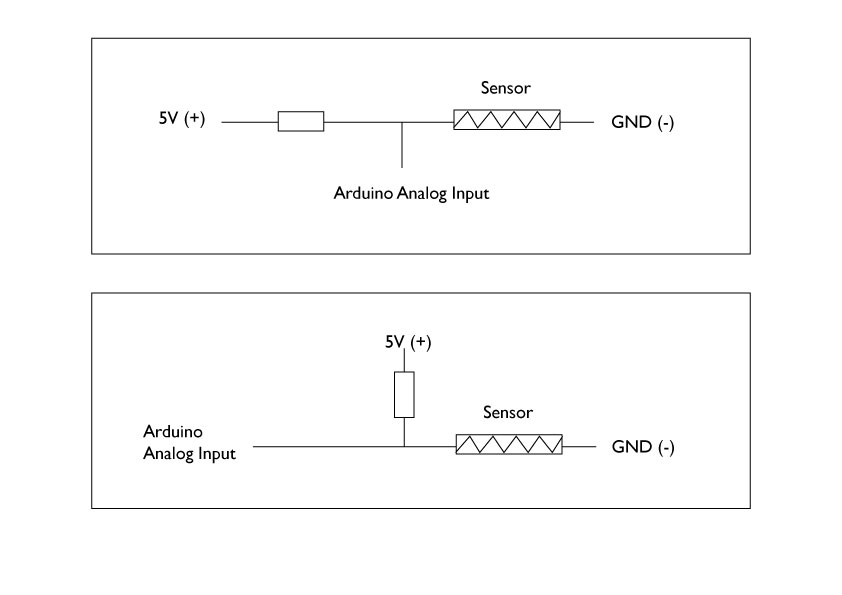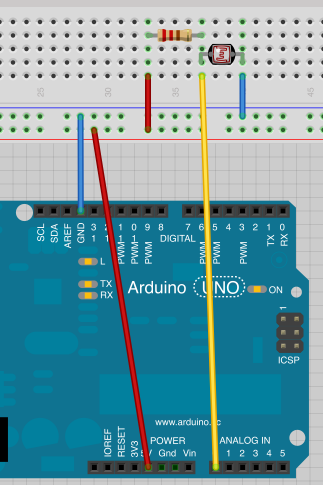# How to connect Analog resistive sensors: Voltage Divider

You can divide the voltage by using 2 resisters.
If you have 2 exactly same resistors, the voltage gets half in the middle, like the first diagram.
As the ratio between two resisters changes, the voltage you get in the middle (between the resisters) changes accordingly.As the analog input pins are reading the voltage input changes, we need to change the voltage that goes into the analog pins by changing the resistance connected to the analog input pin. Potentiometer is acting as voltage divider, between left pin and middle pin as one resister, and the middle pin and right pin as another. As you turn the knob, the ratio of two resisters change, thus the voltage flowing into the middle pin changes.

How to connect analog sensorsHere is an example of photocell connection view on breadboard.